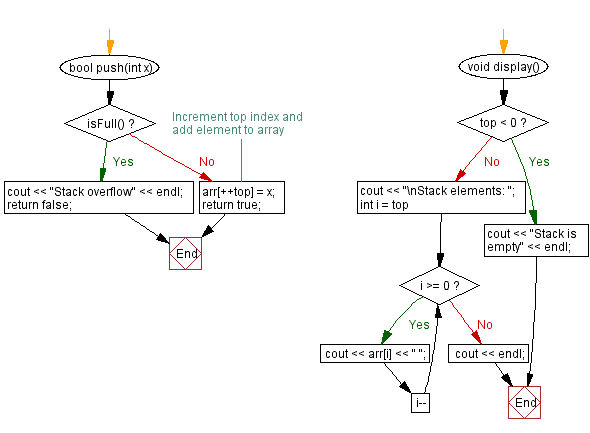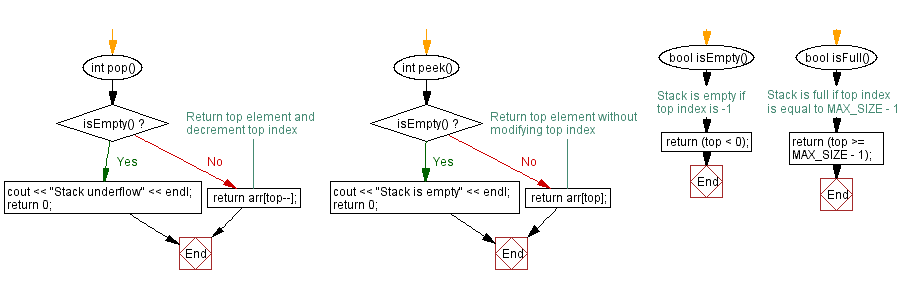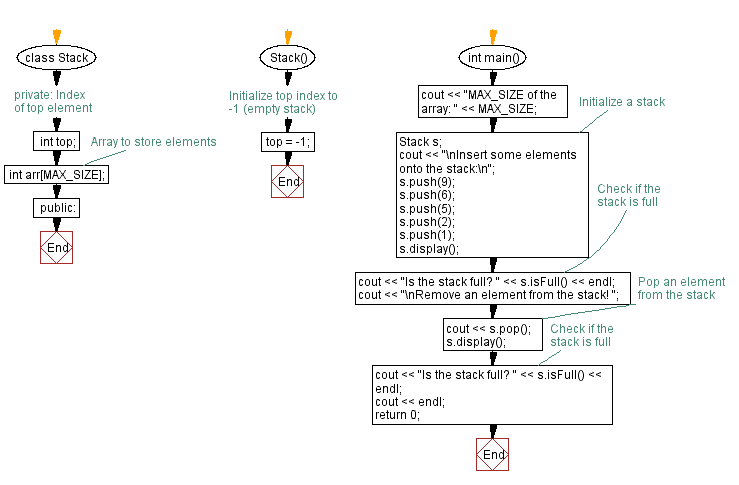﻿ C++ Check if the stack (using an array) is full

# C++ Stack Exercises: Check if the stack (using an array) is full

## C++ Stack: Exercise-2 with Solution

Write a C++ program to implement a stack using an array with push and pop operations. Check if the stack is full.

Test Data:
MAX_SIZE of the array: 5
Insert some elements onto the stack:
Stack elements: 1 2 5 6 9
Is the stack full? 1

Sample Solution:

C++ Code:

``````#include <iostream>

using namespace std;
#define MAX_SIZE 5 // Maximum size of stack
class Stack {
private:
int top; // Index of top element
int arr[MAX_SIZE]; // Array to store elements
public:
Stack() {
top = -1; // Initialize top index to -1 (empty stack)
}

bool push(int x) {
if (isFull()) {
cout << "Stack overflow" << endl;
return false;
}
arr[++top] = x; // Increment top index and add element to array
return true;
}

int pop() {
if (isEmpty()) {
cout << "Stack underflow" << endl;
return 0;
}
}

int peek() {
if (isEmpty()) {
cout << "Stack is empty" << endl;
return 0;
}
}

bool isEmpty() {
return (top < 0); // Stack is empty if top index is -1
}

bool isFull() {
return (top >= MAX_SIZE - 1); // Stack is full if top index is equal to MAX_SIZE - 1
}

void display() {
if (top < 0) {
cout << "Stack is empty" << endl;
return;
}
cout << "\nStack elements: ";
for (int i = top; i >= 0; i--)
cout << arr[i] << " ";
cout << endl;
}
};
int main() {
cout << "MAX_SIZE of the array: " << MAX_SIZE;
//Initialize a stack
Stack s;
cout << "\nInsert some elements onto the stack:\n";
s.push(9);
s.push(6);
s.push(5);
s.push(2);
s.push(1);
s.display();
cout << "Is the stack full? " << s.isFull() << endl; // Check if the stack is full
cout << "\nRemove an element from the stack! ";
cout << s.pop(); // Pop an element from the stack
s.display();
cout << "Is the stack full? " << s.isFull() << endl; // Check if the stack is full
cout << endl;
return 0;
}
``````

Sample Output:

```MAX_SIZE of the array: 5
Insert some elements onto the stack:

Stack elements: 1 2 5 6 9
Is the stack full? 1

Remove an element from the stack! 1
Stack elements: 2 5 6 9
Is the stack full? 0
```

Flowchart:CPP Code Editor: